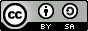### APLIKASI METODE KERNEL DALAM ESTIMASI FUNGSI HAZARD

#### Abstract

Let T be a nonnegative random variable representing the lifetimes of individuals in some population. Let f(t) denote the probability density function of T and F(t) denote the distribution function of T, the hazard function of T defined as
F(t) - 1  S(t)   where
S(t) f(t) h(t)   If equation (1) integrated we have cumulative hazard function H (t).  This paper describes application of kernel method for estimation of hazard function h (.) based censoring data. And then we will show that the hazard estimator is unbiased asymptotically, consistent, and normal asymptotically.

Key word: kernel methods, estimation hazard function.

#### Full Text:

PDF

DOI: http://dx.doi.org/10.18269/jpmipa.v6i2.345

### Refbacks

• There are currently no refbacks.